# Google’s Obsession With Neural Networks

I’ve talked about why Google shifted from a rules-based to machine learning-based engine previously, but I didn’t talk about the details of which exact technology was being used to do that. That’s because the topic of solving “nonlinear classification problems” is an extremely complicated and fascinating story in itself.

With supervised machine learning, humans first assign “labels” and “features” to a set of data. Labels are the thing that is being predicted. In search engine algorithm parlance, it could be as simple as “is this site spammy?”. Features are the inputs. Things like content, links, or really anything that is indexed by a search engine can be a feature. In a modern search engine, there are thousands of features used during the training process. This means that a simple linear equation fails us when trying to compute a solution to their relationships with our labels.

Let’s visualize exactly why these problems are so hard to classify. Here’s a linear problem: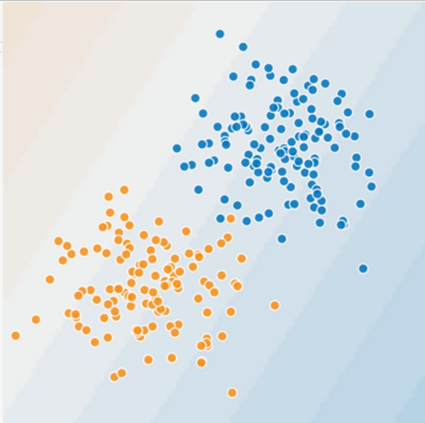The orange and blue dots represent two features of a data set. Let’s call the orange dots “spammy sites” and blue dots “non-spammy sites”. Pretty easy to draw a linear equation to classify. But now let’s look at how the real world looks: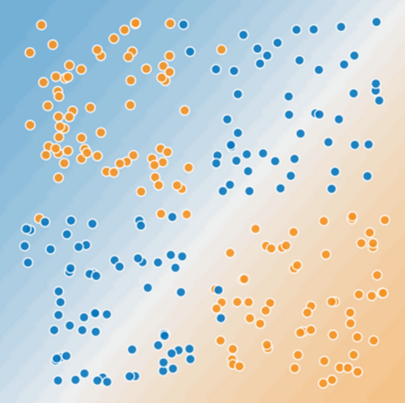Here, there is no straight line that can be used to separate the classifications. This is a nonlinear problem, and a simple one at that. Real classification problems inside of a modern search engine, whether dealing with images or link and content algorithms, often have a graph that represents a random looking chart of blues and oranges (and many other colors).

We have to find the nonlinear equation to solve this problem. A traditional way to do this would be to explicitly determine an equation that produced a curved line such that all of the orange dots were grouped together, and all of the blue dots were grouped together.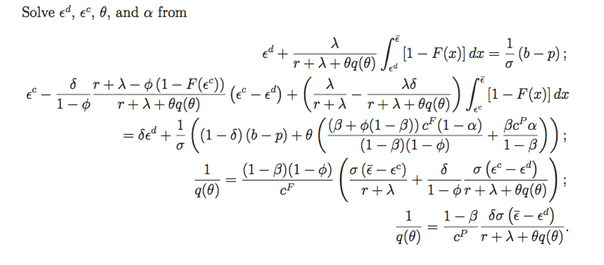In the course of my electrical engineering degree, I of course learned that NAND logic gates could be used to represent ANY equation.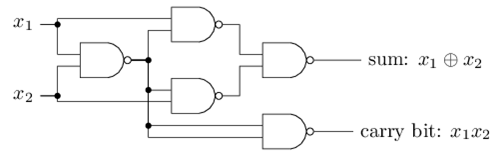What if we could replace the NAND logic gates with a tunable logic gate? Well, we can. They’re called perceptrons: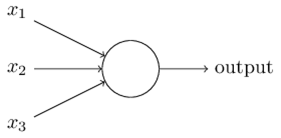Perceptrons, as it turns out, are very simple, like NAND logic gates. They can take various inputs, run a simple conversion, and output the result. We can add weights to the inputs, and this allows us to create “placeholders” so we can later program the circuit after it is already all hooked up: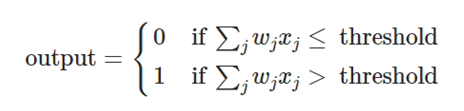What we have done here is the basic building blocks of a neural network. Neural networks are one of the most beautiful programming paradigms ever invented. In the conventional approach to programming, we tell the computer what to do, breaking big problems up into many small, precisely defined tasks that the computer can easily perform. By contrast, in a neural network we don’t tell the computer how to solve our problem. Instead, it learns from observational data, figuring out its own solution to the problem at hand.

It turns out that we can devise learning algorithms which can automatically tune the weights and biases of a network of artificial neurons. This tuning happens in response to external stimuli, without direct intervention by a programmer. These learning algorithms enable us to use artificial neurons in a way which is radically different to conventional logic gates. Instead of explicitly laying out a circuit of NAND and other gates, our neural networks can simply learn to solve problems, sometimes problems where it would be extremely difficult to directly design a conventional circuit.

To solve these complex nonlinear problems, search engines use these neural networks to determine what this nonlinear equation is that represents the relationship between features and labels in its dataset. In reality, neural networks are simply layers of linear decision points that are activated by nonlinear functions. Here is a simple drawing of one: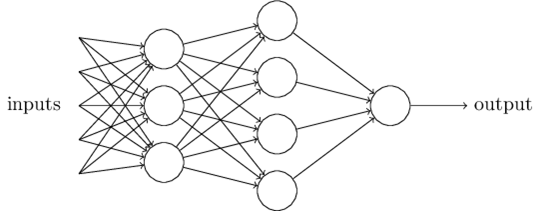By the way, in modern neural networks, we use more subtle logic in our neurons. This is because we want small changes in any neuron to only create small changes in the network output. Perceptrons, by nature, can cause drastic shifts in output (from 0 to 1) by a slight input change. This can cause the machine learning process to become very hard to zone in on the final solution. So, instead, we use things like sigmoid neurons: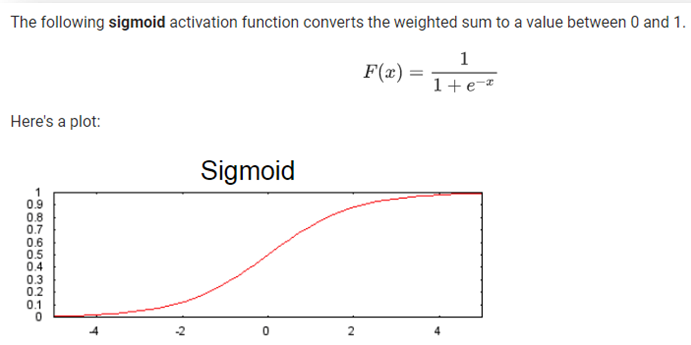The sigmoid neurons allow us to make slight modifications to the weights and biases of individual neurons, during the training process, and learn the relationships between that neuron and the final output of the network. The neural networks can have many layers of neurons, and be connected by many different pathways, often emulating feedback loops that have “memory”.

These neural networks are then fed with training data, and the label values are recorded against a specific target label value. The difference between these values is called the compute loss.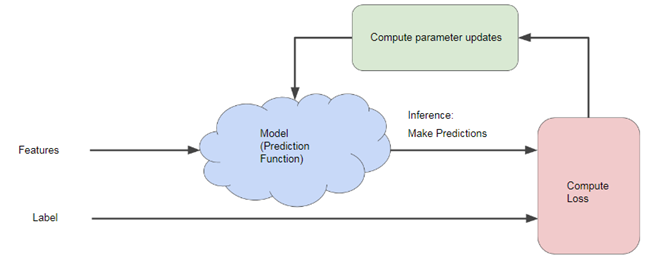As with all machine learning models, for the model to output something useful, its loss factor needs to be minimized. So a process called gradient descent is used to find the minimal loss factor.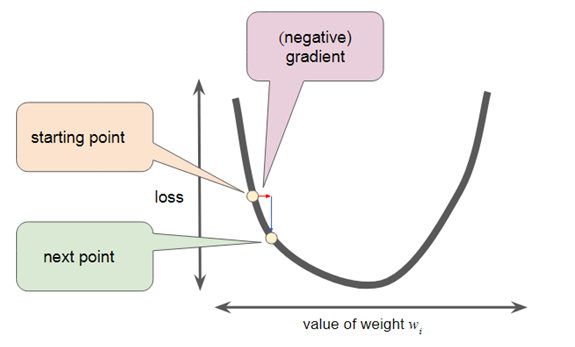This iterative approach calculates a smaller loss each time until a minimum value is found. For extremely large datasets like a modern search engine uses, a more intelligent process called Mini-Batch Stochastic Gradient Descent (Mini-batch SGD) is used.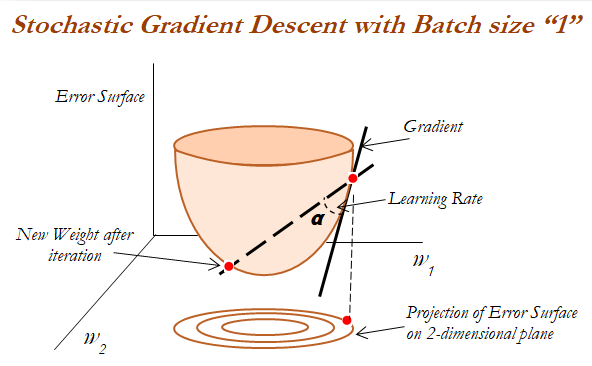Once the predicted output of our network is close to the actual labeled data, we have solved for the equation that we were looking for!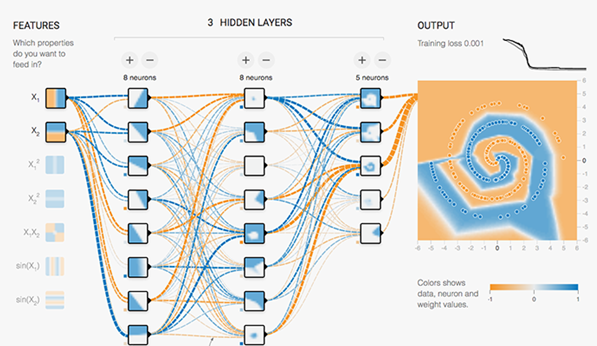Each neuron has been tuned as if we perfectly put together a set of NAND logic gates to emulate the equation that gives us the classification we needed. These neural networks can solve extremely complicated nonlinear problems. Problems that our brains solve very easily, but are a nightmare for a rules-based engine, can be easily classified using this approach. One practical example that is very easy to visualize is the classification of images.Images are a bit trickier in that the features can be in different dimensions and in different orders. Here, a specific implementation of neural networks, called convolutional neural networks, is used. In convolutional neural networks, multilayer neurons are used where each neuron in one layer is connected to many neurons in the next layer. This makes the neural network able to easily assemble more complex patterns using smaller and simpler patterns. These neural networks can look at a piece of an image, in any scale or position, and reliably assign a probability value that the piece belongs to a specific classification.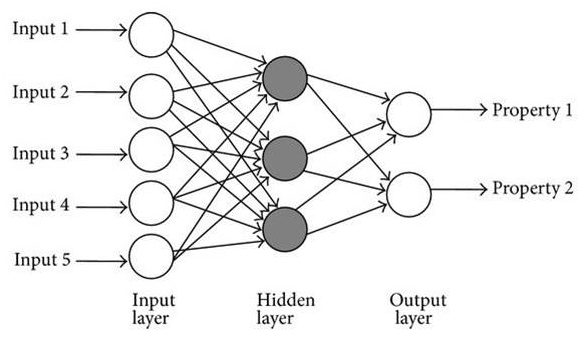One of the biggest challenges in machine learning is the labeling of data. Machine learning needs quality labeled data points, and lots of them! Here, Google ingeniously came up with a way for unsuspecting Internet users to label its data for them, at scale. Some of my fellow alumni at Carnegie Mellon came up with a computerized test to see if a user was a human, primarily to fight comment spam. They called it ReCAPTCHA. Google purchased ReCAPTCHA and eventually used it to solve another problem: labeling image dataIf you’ve used the Internet, you’ve probably used a version of ReCAPTCHA. You’ve been labeling data for Google’s neural networks and you didn’t even know it!

With all of this labeled data, Google and other companies have been able to use this to train their convolutional neural networks to routinely and accurately label all kinds of image data. Other companies have taken this data and expanded into many other industries, including self-driving cars.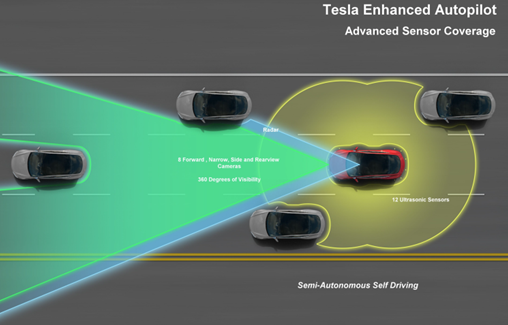What about non-image data? Remember Google’s “Search Quality Rating Guidelines”? Here, it created a playbook for its human “quality raters” to label its data. It then used neural networks to solve the extremely complex nonlinear equations that involved many different algorithms operating on its content and link indexes.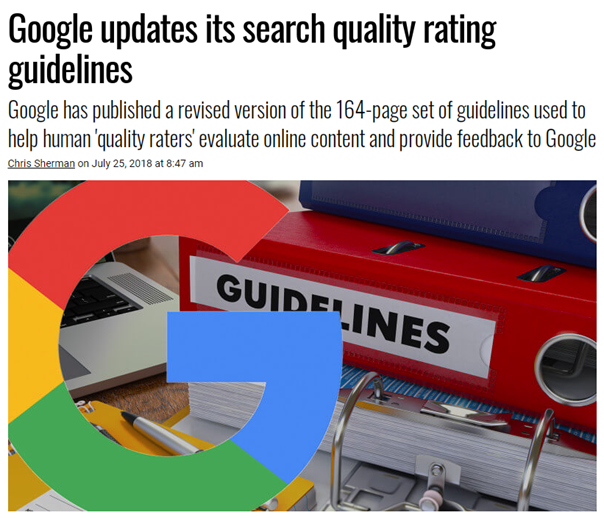The interesting part about neural networks is that even the search engineers at Google don’t understand exactly how they work. Neural networks are essentially black boxes of relationships between their families of link and content scoring algorithms, and the algorithmic scoring outputs that these models can then predict.And that’s great for Google. As we learned in Why Google went from a rules-based to a ML-based search engine, Google is perfectly fine with keeping its operational algorithms as convoluted as possible.